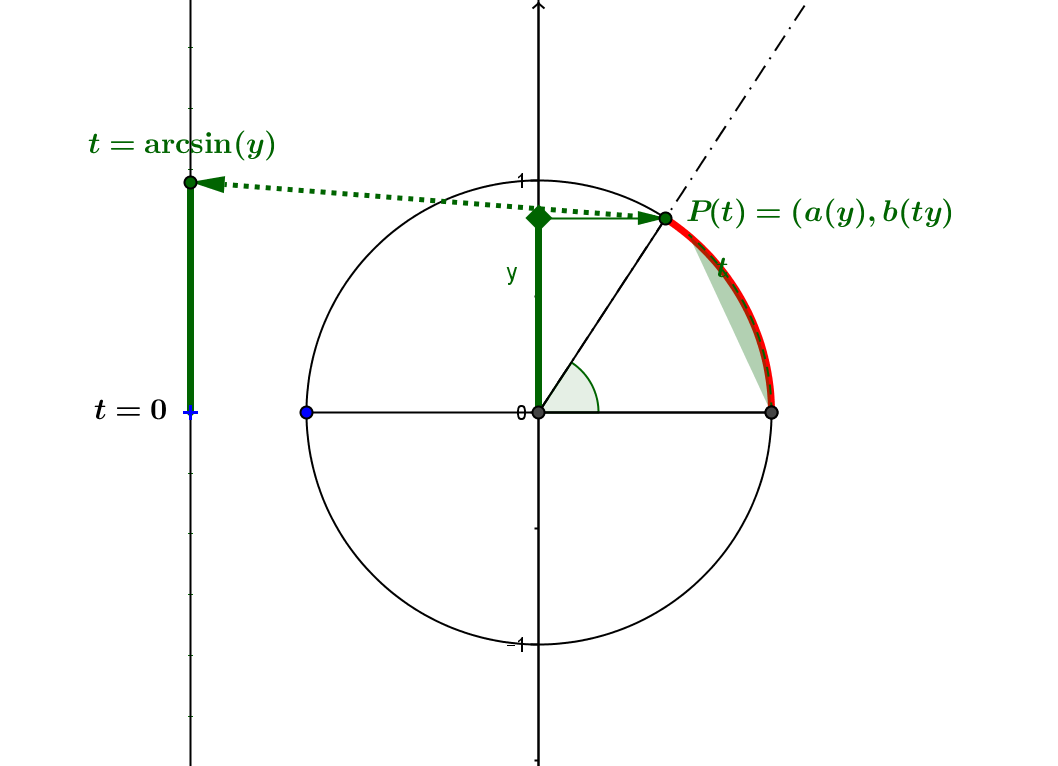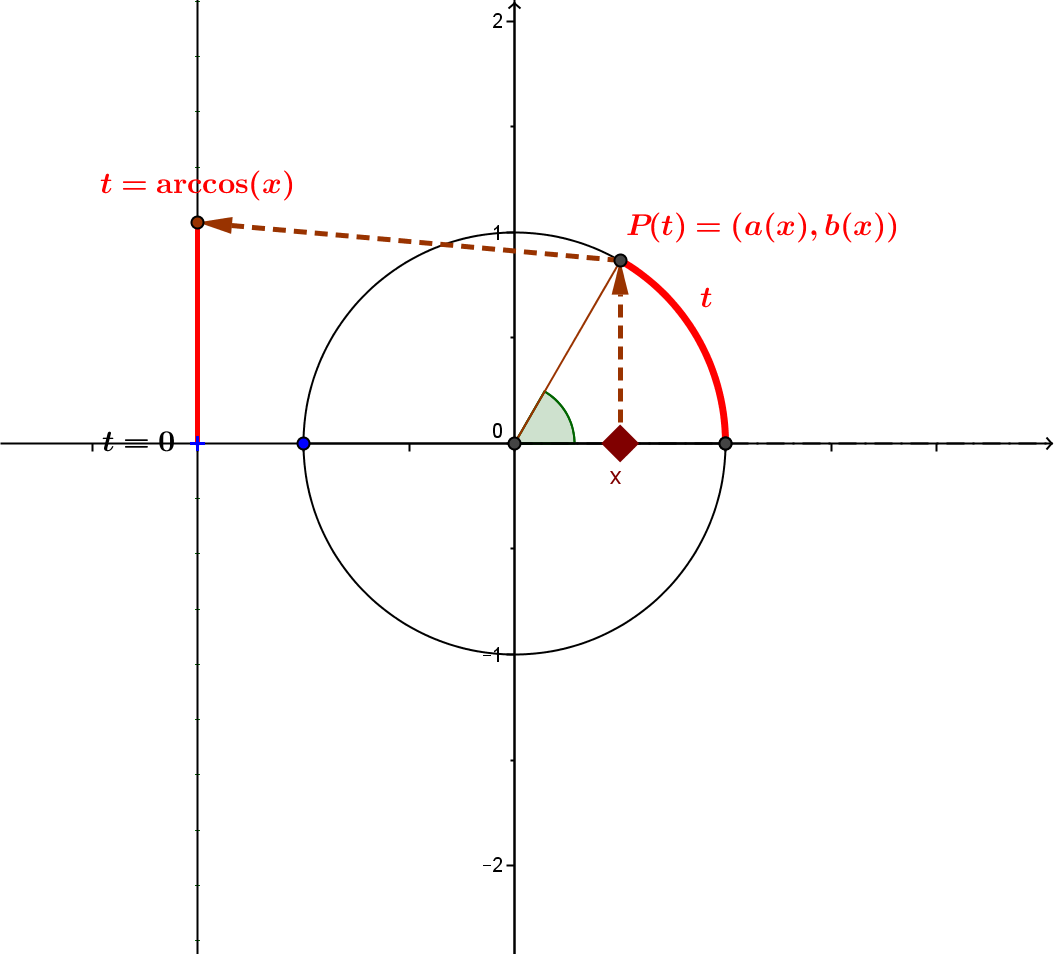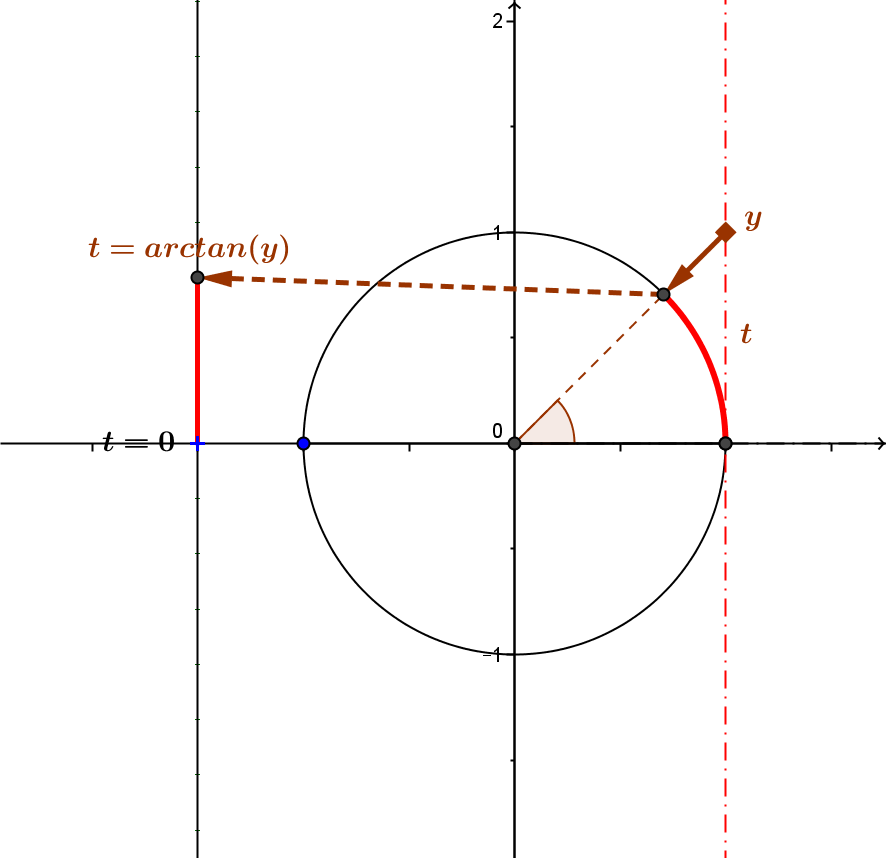TRIG.INV.UC: Inverse Connections to The Unit Circle.

The connection of the inverse trigonometric functions (arcsine, arccosine, and arctangent) to the unit circle is made by reversing the unit circle definitions  of sine, cosine, and tangent using "the wrapping function". $P: t \to P(t)= (x(t),y(t))$ restricted to particular intervals $[-\pi/2.\pi/2], [0,\pi]$ and  $(-\pi/2, \pi/2)$.
Find the appropriate corresponding point on the unit circle:
 Step Arcsine Arccosine Arctangent Locate the input variable value on the appropriate axis in the plane of the unit circle: Use $(0,y)$ on the vertical axis of the unit circle plane Use $(x,0)$ on the horizontal axis of the unit circle plane. Use $(1,y)$ on the axis $x =1$ in the unit circle plane. Reverse the appropriate projection to find a unique point $P(t)$ on the unit circle. Project parallel to the horizontal axis to a point $P(t) = (a(y),b(y))$ on the unit circle with $a(y) \ge 0$. Project parallel to the vertical axis to a point $P(t) = (a(x),b(x))$ on the unit circle with $b(x) \ge 0$. Project toward the point $O =(0,0)$ to a point $P(t) = (a(y),b(y))$ on the unit circle with $a(y) \gt 0$. Find the measure $t$ of the arc on the unit circle in the appropriate interval so that $P(t) = (a,b)$ The measure $t$ of the arc on the unit circle is the arcsine  of $y$,: $t = \arcsin(y)$ The measure $t$ of the arc on the unit circle is the arccosine  of $x$,: $t = \arccos(x)$ The measure $t$ of the arc on the unit circle is the arctangent  of $y$: $t = \arctan(y)$ Unit Circle DiagramConnections to The Unit Circle of Arcsine, Arccosine, and Arctangent: Three GeoGebra Mapping Diagrams
 arcsine arccosine arctangent

Notice how the mapping diagram values are connected to measurements related to the unit circle by considering the line from the origin $O = (0,0)$ and the point $P(t) = (x(t), y(t))$ determining the value of $t$ by measuring the appropriate arc on the unit circle.

Use the point on the appropriate axis to control $P(t)$ and thus the angle measured in radians or degrees.